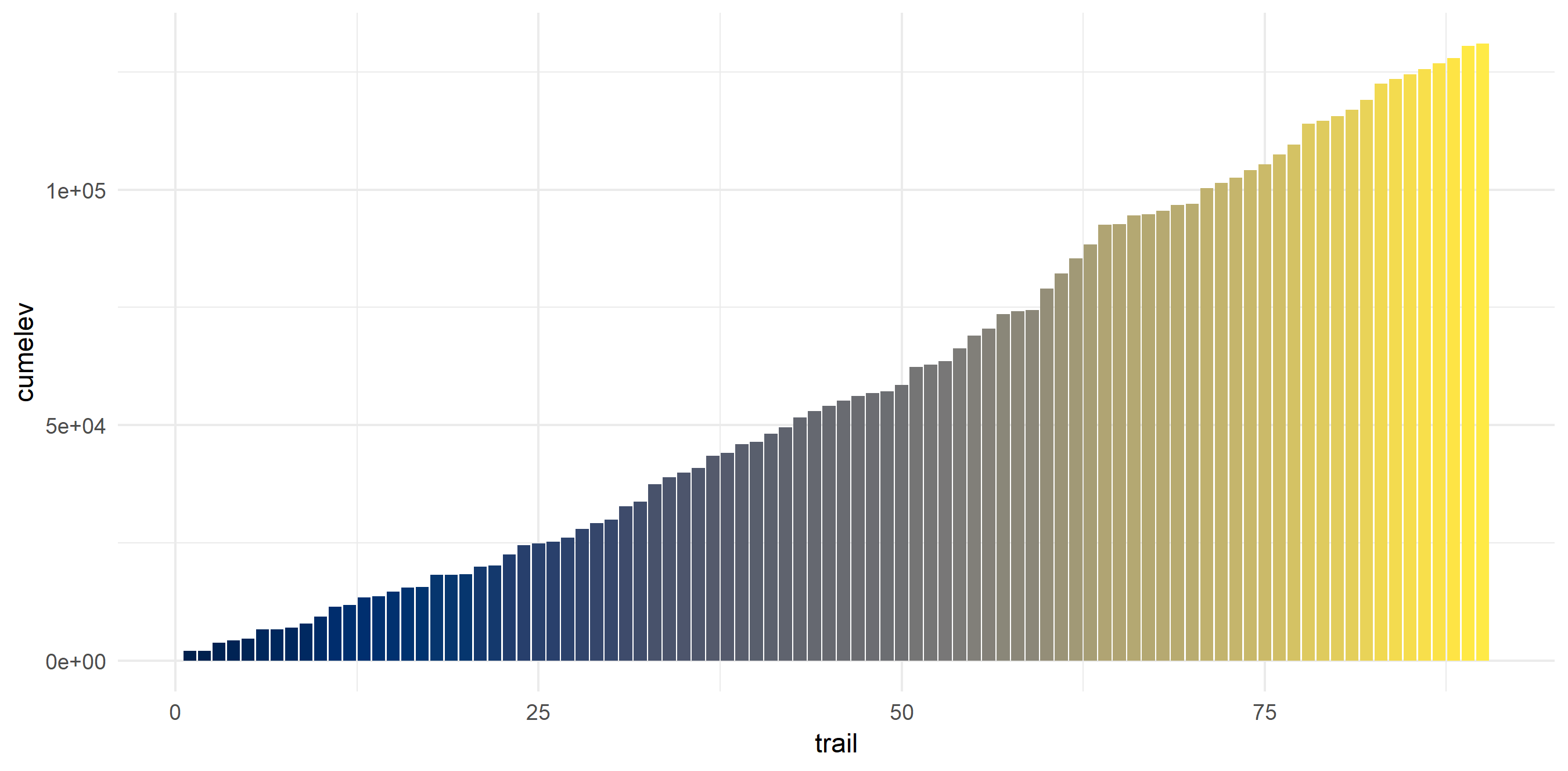# Taking a peek into my hiking data

import
visualize
analyze
I got my hands on a bunch of GPX files.
Published

April 6, 2019

I moved to Seattle at the end of 2016 and since then have done over 100 hikes (depending on your definition of ‘a hike’). I must admit I’ve been abysmal at tracking any data regarding my hiking activity beyond a Google spreadsheet, despite the ubiquity of trail tracking apps that exist.

Recently, I signed up on AllTrails to start collecting data on my hikes. The Pro service offers many wonderful features, including the ability to download GPX data on hikes. I was so excited by this that I decided to try to visualize the hikes I have done.

I ran a poll on Twitter in which I asked whether people embed code in the main text of their blog post or at the end. 91% embed in the main text [n = 85]. I structured this post accordingly.

## Pulling data

### Choose packages

It took a bit to decide which packages had the functions needed to run the spatial analyses. In the end, I decided on:

• plotKML: A package containing functions to read GPX files.
• geosphere: A package containing functions for geospatial calculations. I decided to use this for finding out distances between lon/lat.
• googleway: A package allowing access to the Google Maps API. To run this, you need to obtain a Google Maps API key and load it to R by using `set_key()`. I use this for elevation calculations but the API can also obtain the distance between points.
``````library(tidyverse)
library(plotKML)
library(geosphere)

### Tidy data

I downloaded each GPX file from AllTrails and saved them in a file in my project organization. Their file names were TRAILNAME.gpx.

• Using `plotKML::readGPX()` results in the files being loaded as lists.
• I used `purrr` in conjunction with `plotKML()` to handily read them in and add the file name to the list.
``````# find gpx files
data_path <-
here::here("data", "raw", "gpx_files")

files <-
dir(data_path, pattern = "*.gpx", full.names = TRUE)

# get trail names
names <-
dir(data_path, pattern = "*.gpx", full.names = FALSE) %>%
str_extract(".+?(?=.gpx)")

gpx_dat <-
map2(files,
names,
bounds = TRUE,
waypoints = TRUE,
tracks = TRUE,
routes = TRUE) %>%
list_modify(trail = .y)) # otherwise you can't tell which entry is for which trail``````

### Calculate elevation

We can use `googleway::google_elevation()` to access the Google Elevation API and calculate elevation for every lon/lat pair from the GPX files. Unfortunately, the API accepts and returns only a few requests at a time (~200 rows for these files). We have over 51,000 rows of data. So, we can create groups for every 200 rows and use a loop to make a call for each

This results in a list, so we can then create a tibble pulling out the data we want.

``````lonlat_dat <-
gpx_dat %>%
map_df(., ~.x\$"routes"[], .id = "trail") %>%
select(trail, lon, lat) %>%
group_by(trail) %>%
ungroup() %>%
mutate(group_number = (1:nrow(.) %/% 200) + 1) # https://stackoverflow.com/questions/32078578/how-to-group-by-every-7-rows-and-aggregate-those-7-values-by-median

dat_lapply <- lapply(1:max(lonlat_dat\$group_number), function(x) {
Sys.sleep(3)

lonlat_dat %>%
filter(group_number == x) %>% # added a filter so you only pull a subset of the data.
do(elev_dat =
data.frame(
df_locations = dplyr::select(., lon, lat),
location_type = "individual",
simplify = TRUE)))
})

dat_lapply_elev_dat <-
dat_lapply %>%
map(., ~ .x\$"elev_dat"[])

elev_df <-
dat_lapply_elev_dat %>% {
tibble(
elevation = map(., ~ .x\$"results.elevation"),
lon = map(., ~ .x\$"results.location"[["lng"]]),
lat = map(.,  ~ .x\$"results.location"[["lat"]])
)
} %>%
unnest(.id = "group_number") %>%
select(group_number, elevation, lon, lat)``````

### Calculate distance

Now we have a list of trails, longitudes and latitudes along their paths, and the elevation for each of those points. Now we want to calculate the distance along the paths.

• We bring back `lonlat_dat` so we know what trails with which each point are associated.
• To use calculate distance, we can use `distHaversine()` with two sets of lon/lat. We create the second set of lon/lat by creating a new variable that takes the “next” value in a vector (so we’re calculating the distance between point A and point B, point B to point C, and so on).
• `cumsum()` accumulates the distances between each set of lon/lat.
• Finally, we calculate the elevation gain for each hike.
``````hiking_dat <-
plyr::join(elev_df, lonlat_dat, type = "left", match = "first") %>%
group_by(trail) %>%
mutate(elev_feet = elevation * 3.281, # to convert to feet
ungroup() %>%
mutate(dist = distHaversine(hiking_dat[, 2:3], hiking_dat[, 7:8])/1609.344) %>% # to convert to miles
group_by(trail) %>%
mutate(cumdist = cumsum(dist),
elev_gain = elev_feet - first(elev_feet)) %>%
ungroup()``````

For nerdy kicks, I also wanted to find out my ‘average’ hike - that is, the average distance, the average elevation, and the average elevation for each distance. I also wanted to see the total distance and elevation for each trail for which I pulled data.

``````avg_elev <- # average elevation by distance
hiking_dat %>%
group_by(round(cumdist, 1)) %>%
summarize(mean(elev_gain))

hiking_dat_by_trail <- # total gain/distance by trail
hiking_dat %>%
select(trail, cumdist, elev_gain) %>%
group_by(trail) %>%
summarize(tot_dist = max(cumdist, na.rm = T),
tot_elev_gain = max(elev_gain)) %>%
mutate(tot_dist_scaled = scale(tot_dist), # for cluster analysis
tot_elev_scaled = scale(tot_elev_gain))``````

## Disclaimer

For data collection, I downloaded each trail’s GPX files from AllTrails. Because these data are proprietary, I will not be providing them. Some things to note:

• Because these are data pulled from the website, they are not indicative of my actual hiking path (for example, Franklin Falls is a 2-mile hike in the summer, but in the winter is a 6-mile snowshoe).
• There are hikes that I did back-to-back that I’d consider one hike but the trails might be listed separately on the site. For example, Deception Pass is actually made up of three small loops.

## The hikes are wide and varied

Being fortunate enough to live near multiple mountain ranges, the hikes come in all shapes and sizes.

``````ggplot() +
geom_density_ridges(data = na.omit(hiking_dat),
aes(x = cumdist,
y = trail,
group = trail),
fill = "#00204c",
rel_min_height = 0.01
) +
theme_minimal() +
theme(legend.position = "none")``````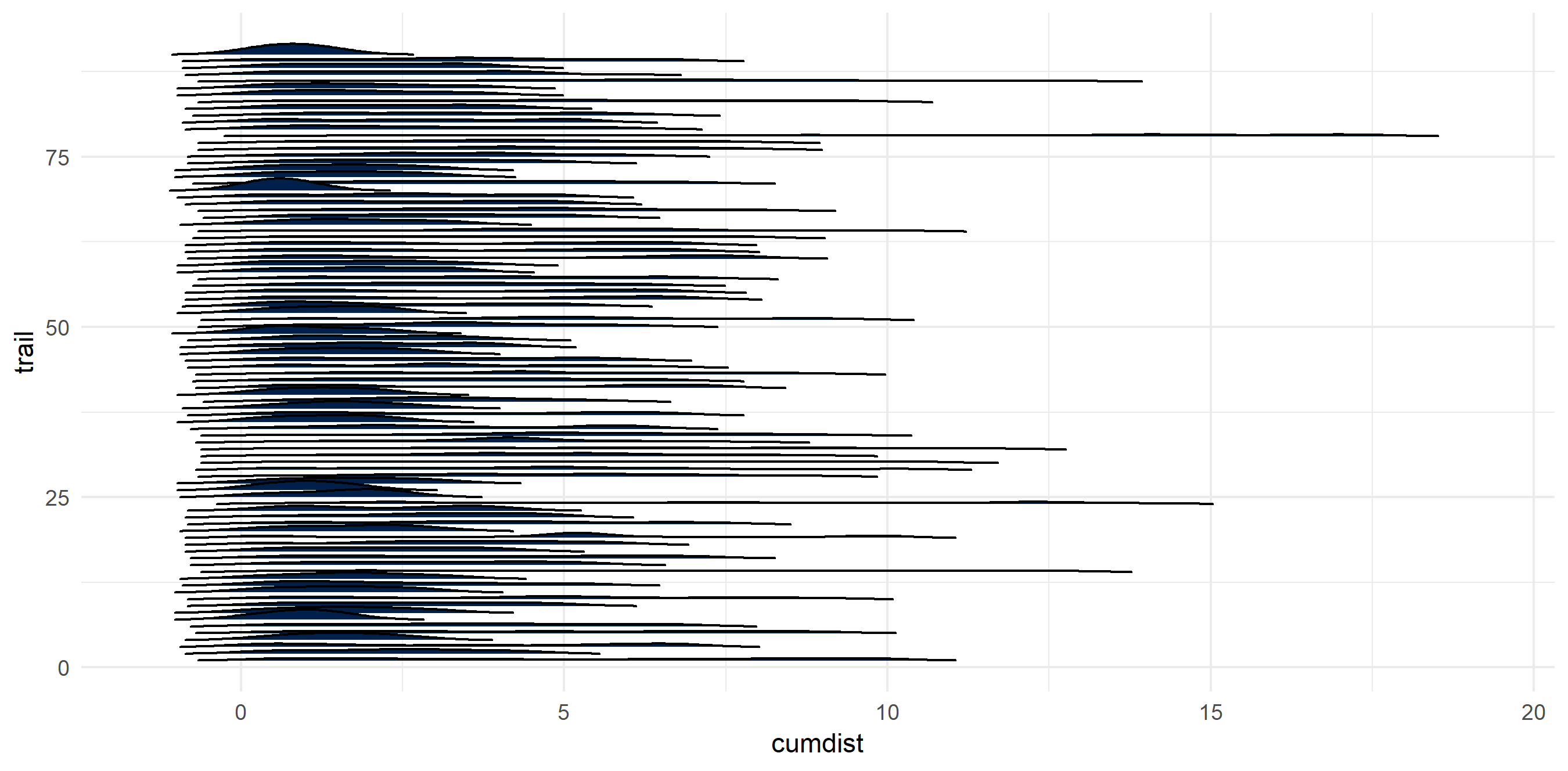I calculated my ‘average hike’ - that is, the average elevation given the cumulative distance traveled.

``````ggplot() +
geom_ridgeline(data = hiking_dat,
aes(x = cumdist,
y = trail,
group = trail,
height = elev_gain),
color = "#c9b869",
alpha = 0) +
geom_line(data = avg_elev,
aes(x = `round(cumdist, 1)`,
y = `mean(elev_gain)`),
color = "#00204c",
size = 2) +
scale_x_continuous(name = "Cumulative Distance (miles)") +
scale_y_continuous(name = "Cumulative Elevation (ft)", limits = c(0, 5000)) +
theme_minimal() +
theme(legend.position = "none")``````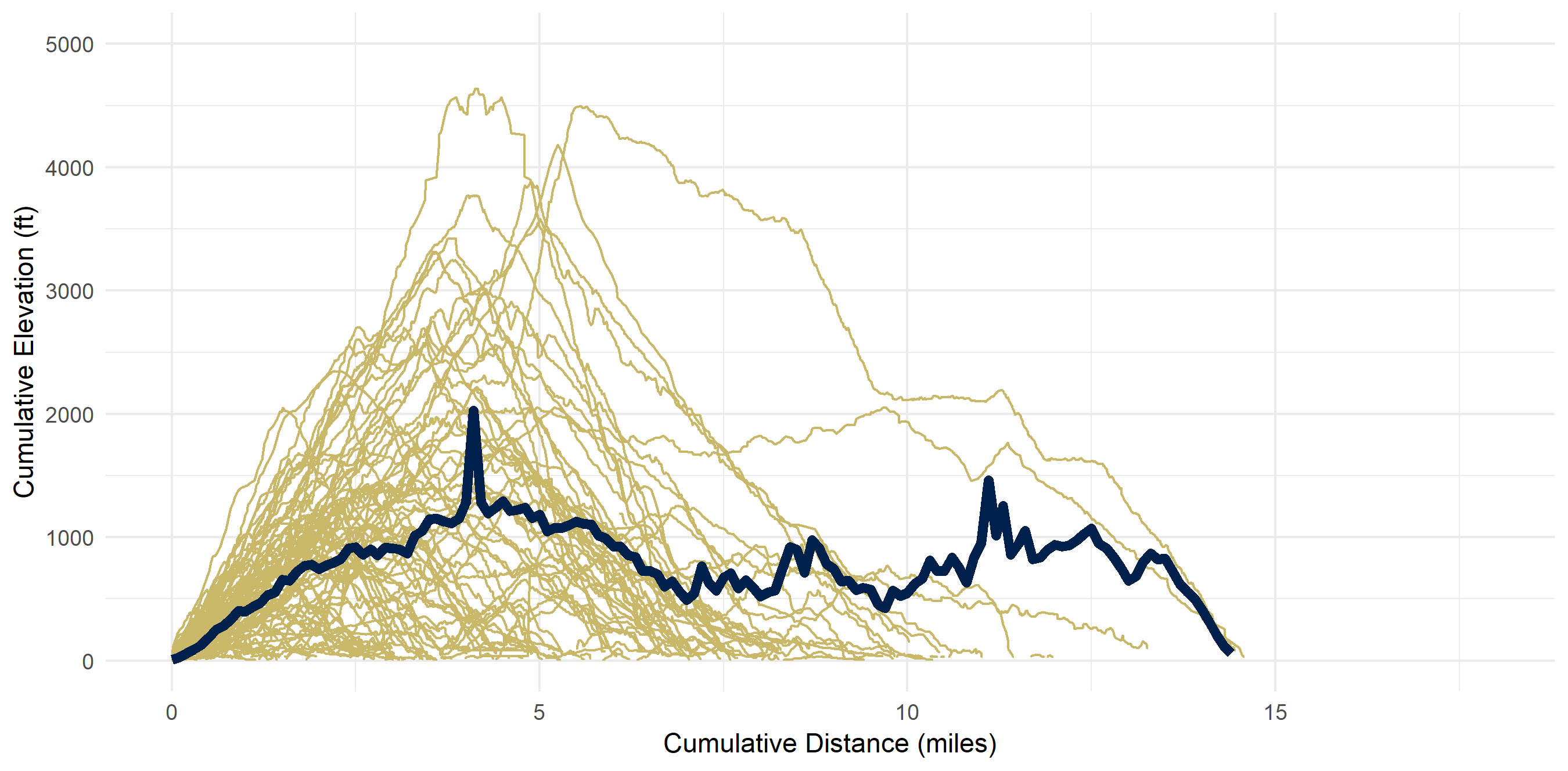## Aggregated Data by Trail

In the aggregate, there seems to be a correlation (r^2 = 0.48) between total distance and total elevation.

``````ggplot() +
geom_point(data = hiking_dat_by_trail,
aes(x = tot_dist,
y = tot_elev_gain,
color = tot_elev_gain,
size = tot_dist)) +
scale_x_continuous(name = "Total Distance (miles)") +
scale_y_continuous(name = "Total Elevation (ft)") +
scale_color_viridis(option = "cividis") +
theme_minimal() +
theme(legend.position = "none")``````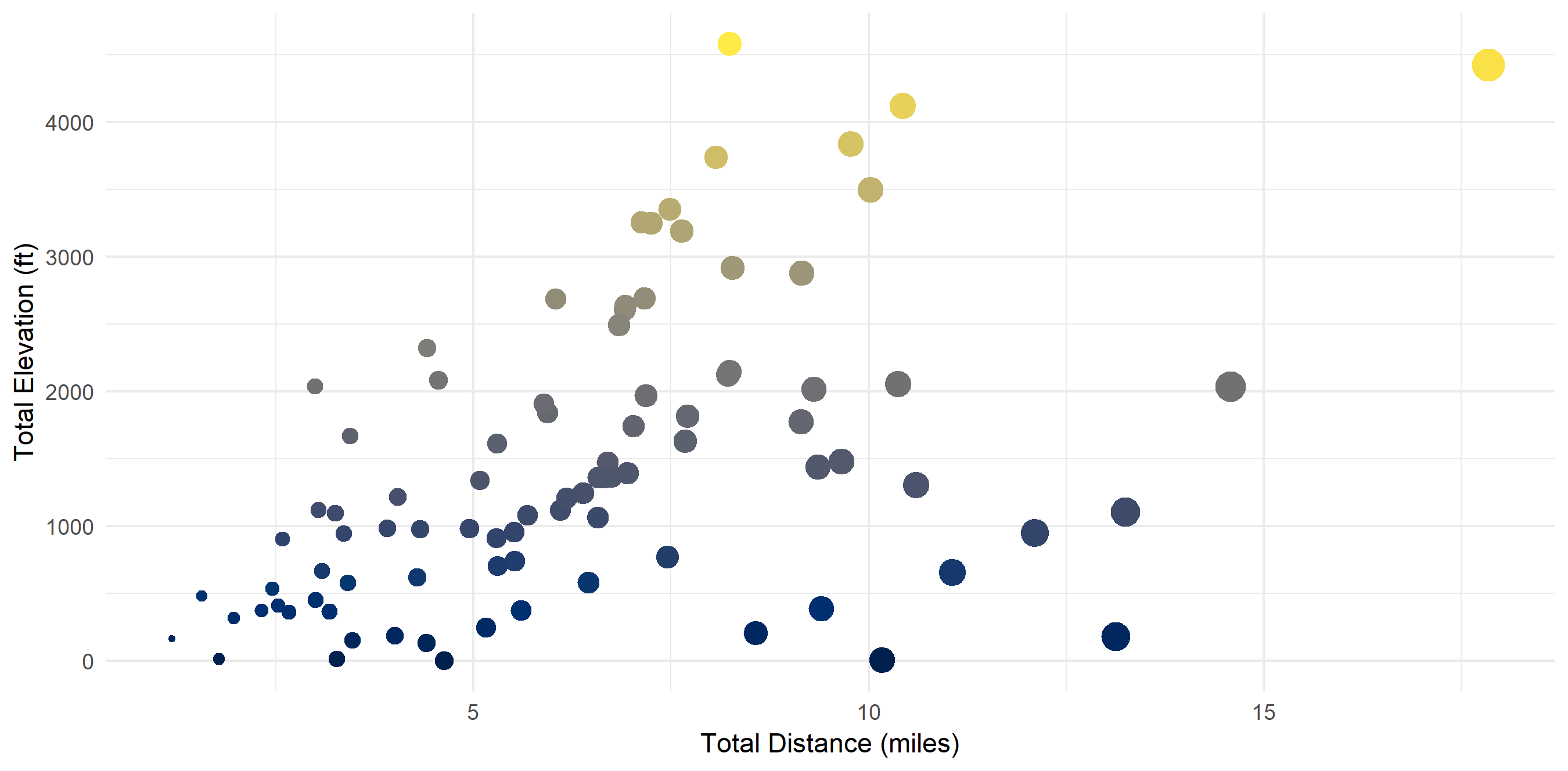## There exist “clusters” of hikes

I ran a quick cluster analysis to see if I can categorize my hikes in any way. Code is in the Methodology section. Four clusters seemed to be optimal. I have dubbed them:

• Cluster 1: “Let’s Get This Over With” (steep & hard)
• Cluster 2: “Easy Peasy Lemon Squeezy” (short & flat)
• Cluster 3: “The Sweet Spot” (not too long, not too high)
• Cluster 4: “I Don’t Care About My Knees Anyway” (too long for my own good)
``````fviz_nbclust(hiking_dat_by_trail[, 4:5], kmeans, method = "wss") # finding optimal number of clusters
k4 <- kmeans(hiking_dat_by_trail[, 4:5], centers = 4, nstart = 25) # calculating clusters

fviz_cluster(k4, data = hiking_dat_by_trail)  +
scale_x_continuous(name = "Scaled Total Distance (miles)") +
scale_y_continuous(name = "Scaled Total Elevation (ft)") +
scale_color_viridis(option = "cividis", discrete = T) +
scale_fill_viridis(option = "cividis", discrete = T) +
theme_minimal()``````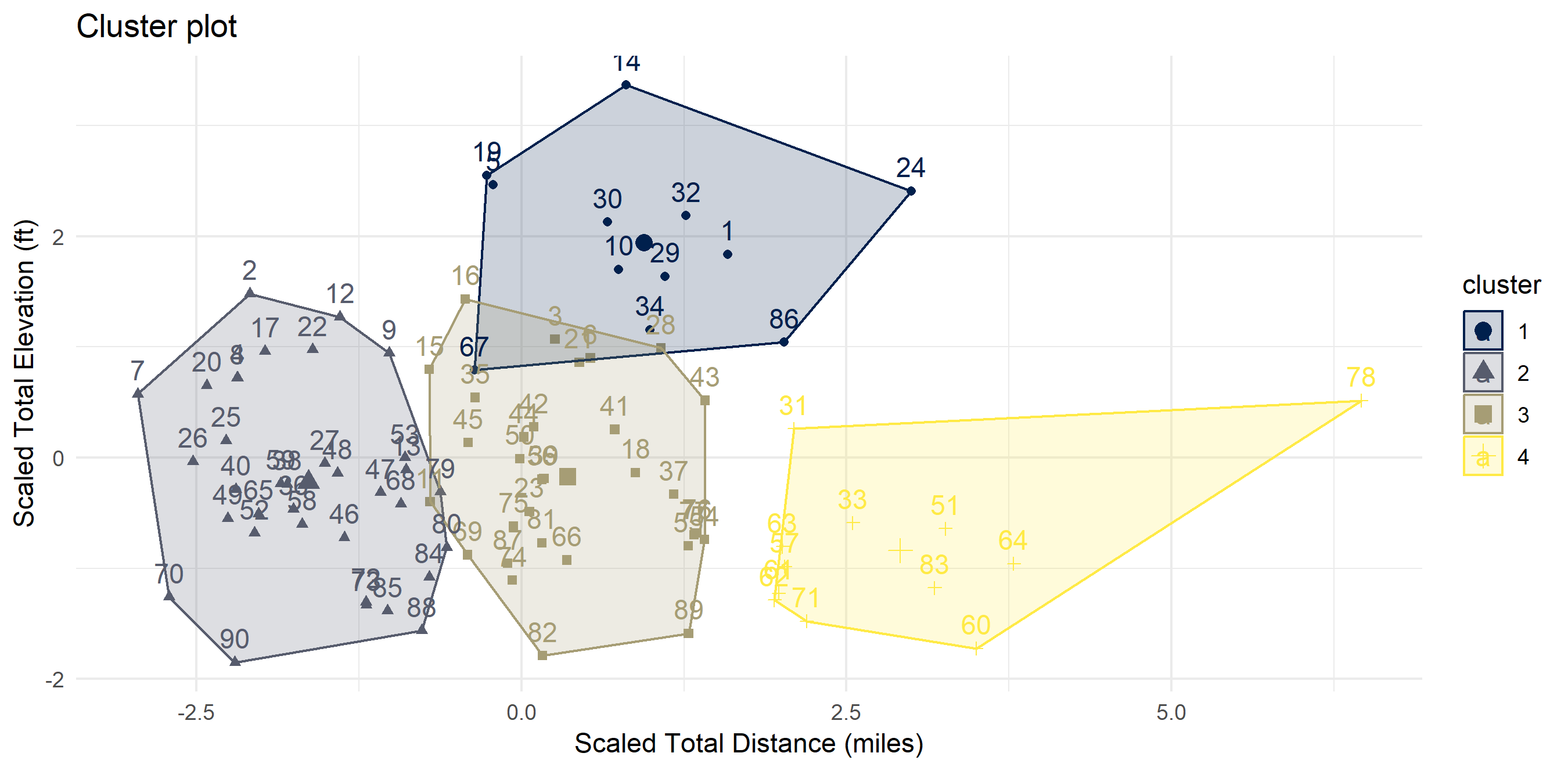## I don’t particularly love long hikes

My average hike is 6.4 miles - and most of them are concentrated around that distance. This makes sense as I usually day hike and need to get back at a reasonable time. My shortest hike was 1.18 miles and my longest was 17.85 (the Enchantments…). In these 90 hikes, I hiked around 576 miles.

``````hiking_dat_by_trail %>%
ggplot(aes(x = tot_dist)) +
geom_histogram(fill = "#00204c") +
xlab("Trail Total Distance (miles)") +
ylab("Count") +
scale_fill_viridis(option = "cividis") +
theme_minimal() +
theme(legend.position = "none")``````
``````hiking_dat_by_trail %>%
mutate(cumdist = cumsum(tot_dist)) %>%
ggplot(aes(x = trail,
y = cumdist,
fill = cumdist)) +
geom_bar(stat = "identity") +
scale_fill_viridis(option = "cividis") +
theme_minimal() +
theme(legend.position = "none")``````

## I don’t dislike high elevation hikes though

Elevation on these hikes ranged from ~0 feet to 4580 feet gain. I averaged 1455.4 feet gain and have climbed 130,984 feet (~24 miles!).

``````hiking_dat_by_trail %>%
ggplot(aes(x = tot_elev_gain)) +
geom_histogram(fill = "#00204c") +
xlab("Trail Total Elevation (ft)") +
ylab("Count") +
scale_fill_viridis(option = "cividis") +
theme_minimal() +
theme(legend.position = "none")``````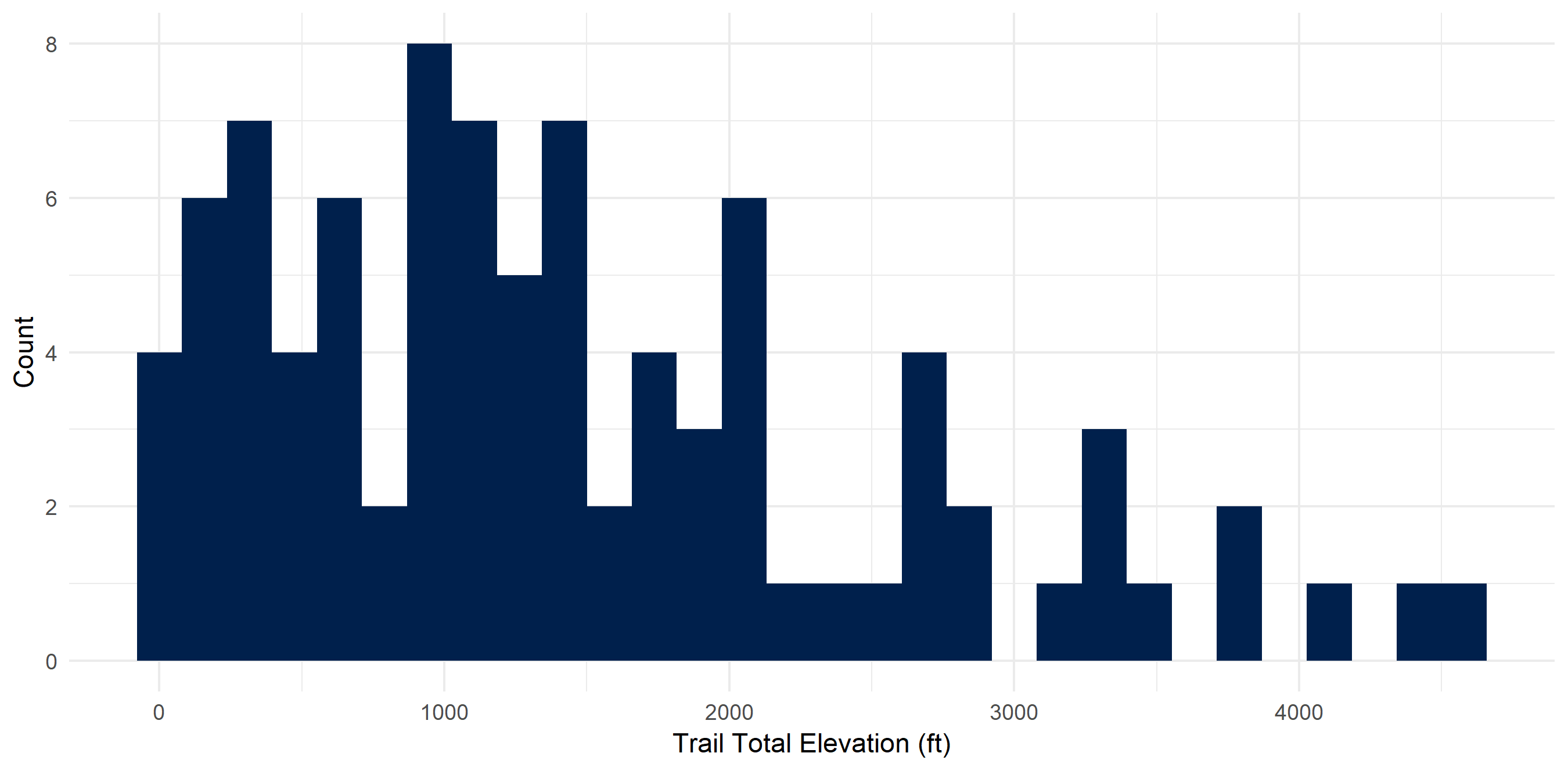``````hiking_dat_by_trail %>%
mutate(cumelev = cumsum(tot_elev_gain)) %>%
ggplot(aes(x = trail,
y = cumelev,
fill = cumelev)) +
geom_bar(stat = "identity") +
scale_fill_viridis(option = "cividis") +
theme_minimal() +
theme(legend.position = "none")``````